一个计算Java问题，求解

JC_404 发布于 2012/02/29 12:55

年薪达不到25W大数据工程师、拿不到Offer全额退款->>>new BigDecimal(38.80).multiply(new BigDecimal(100)).subtract(new BigDecimal(1)).longValue()

(long)(38.80*100-1)

Java算出来的结果都是:3878

以下是问题补充：

@JC_404：感动得泪流满面，本来只是想解决问题而已，结果连前因后果都刨出来了 (2012/02/29 22:20)

1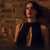new BigDecimal("38.80").multiply(new BigDecimal(“100”)).subtract(new BigDecimal(“1”)).setScale(0)

1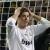0

.floatValue()

0
b
new BigDecimal("38.80").multiply(new BigDecimal(100)).subtract(new BigDecimal(1)).longValue()
0
b
new BigDecimal 一定要用字符串形式，不要用基本类型，详细请参考api
0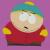0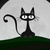引用来自“田波”的答案

new BigDecimal 一定要用字符串形式，不要用基本类型，详细请参考api
new BigDecimal("38.80").multiply(new BigDecimal("100"))
.subtract(new BigDecimal("1")).longValue();

0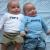longValue

public long longValue()
Converts this BigDecimal to a long. This conversion is analogous to a  narrowing primitive conversion from double to short as defined in the  Java Language Specification: any fractional part of this   BigDecimal   will be discarded,